ICSE Class 10 Maths Sample Question Paper – Mock 5 with Answers can be accessed at BYJU’S. The detailed explanations of these questions are available in a downloadable pdf format, so that students can refer to them, whenever required. They can also download sample papers for class 10 maths in pdf format to practise for the board examination.

Though Maths is difficult for the students, they can easily score maximum marks in the ICSE Class 10 Annual exam through continuous practice. Practising different types of questions and papers will help the students to excel in their problem solving skills. To help the students in their preparation, BYJU’S has provided important questions of ICSE Class 10 Maths here.

#### ICSE Class 10 Maths Mock Sample Paper 5 with Solutions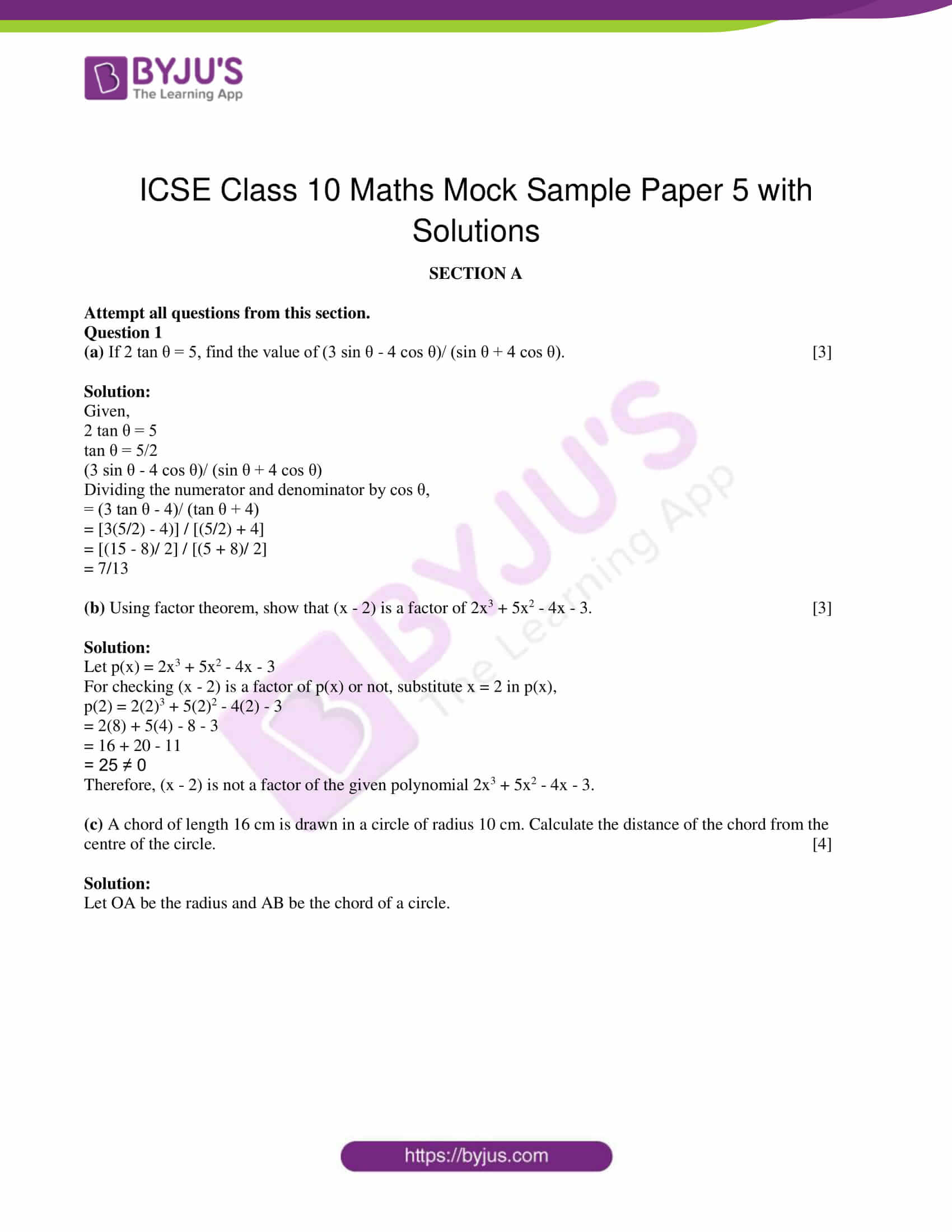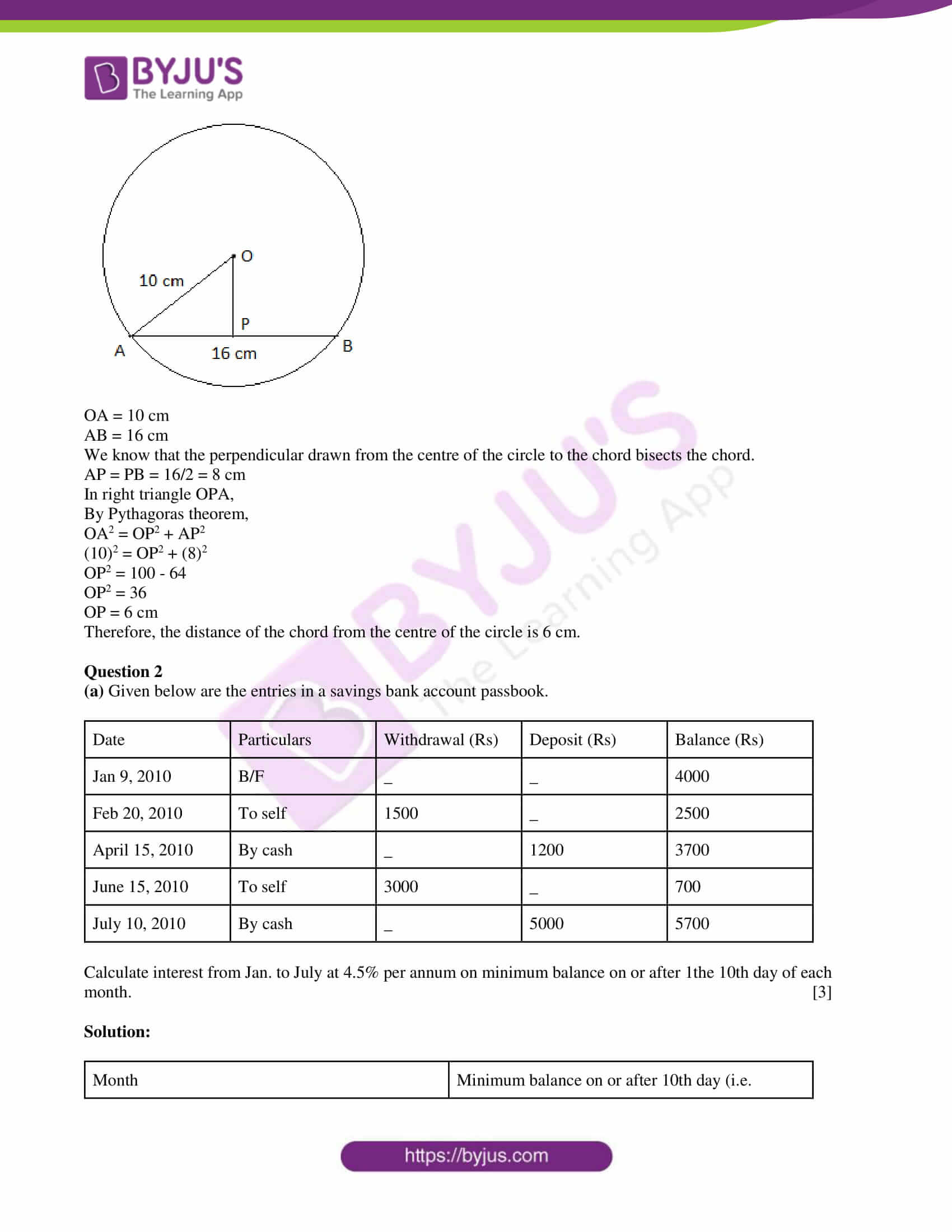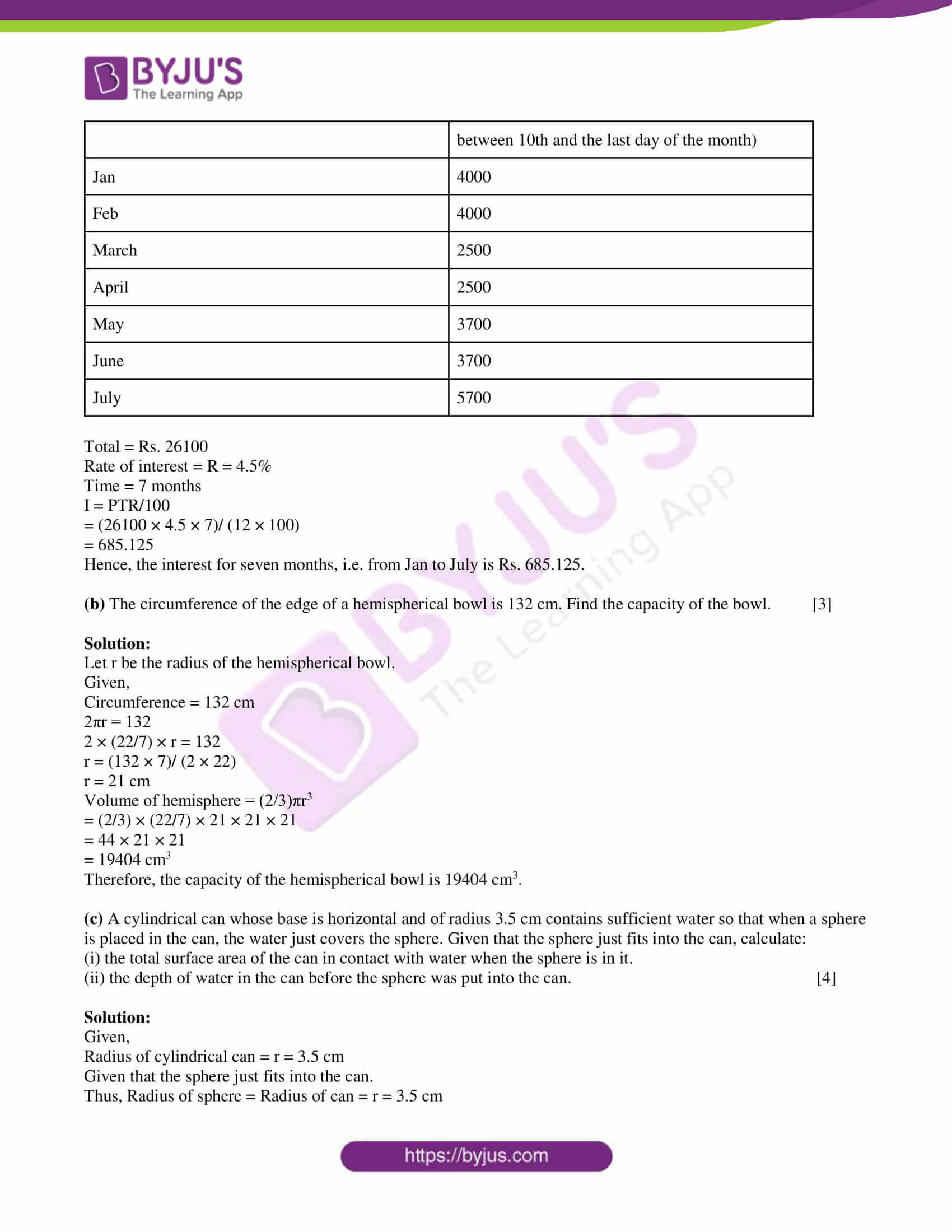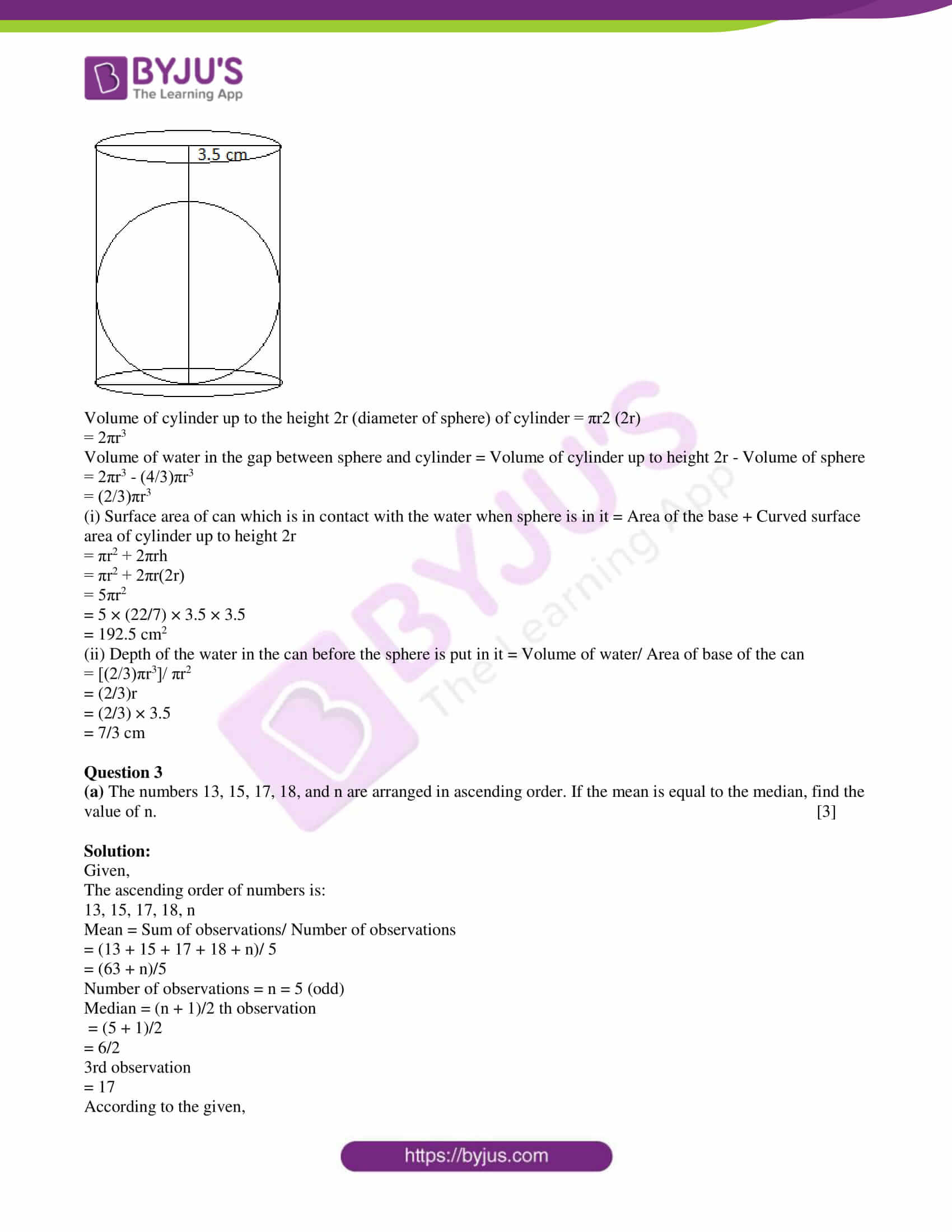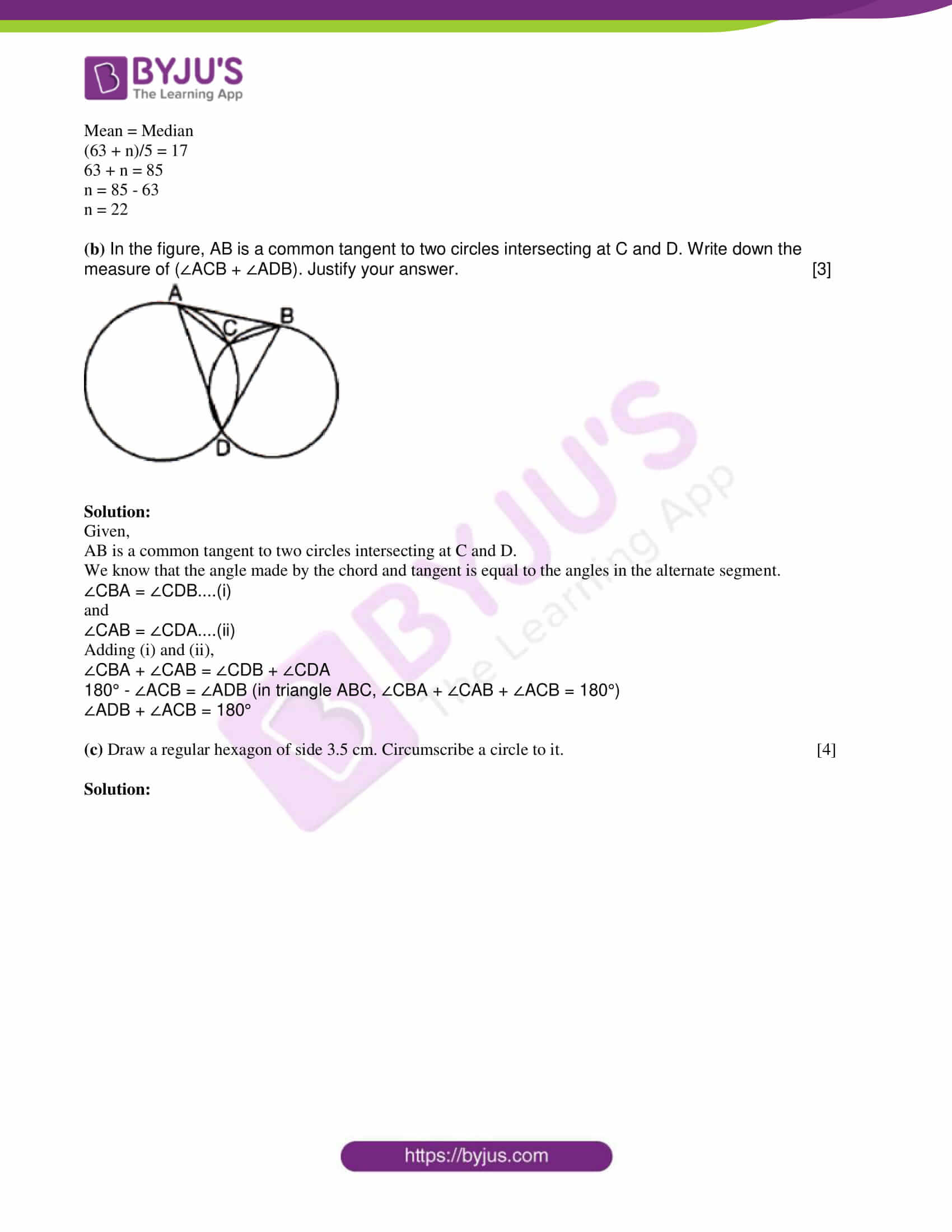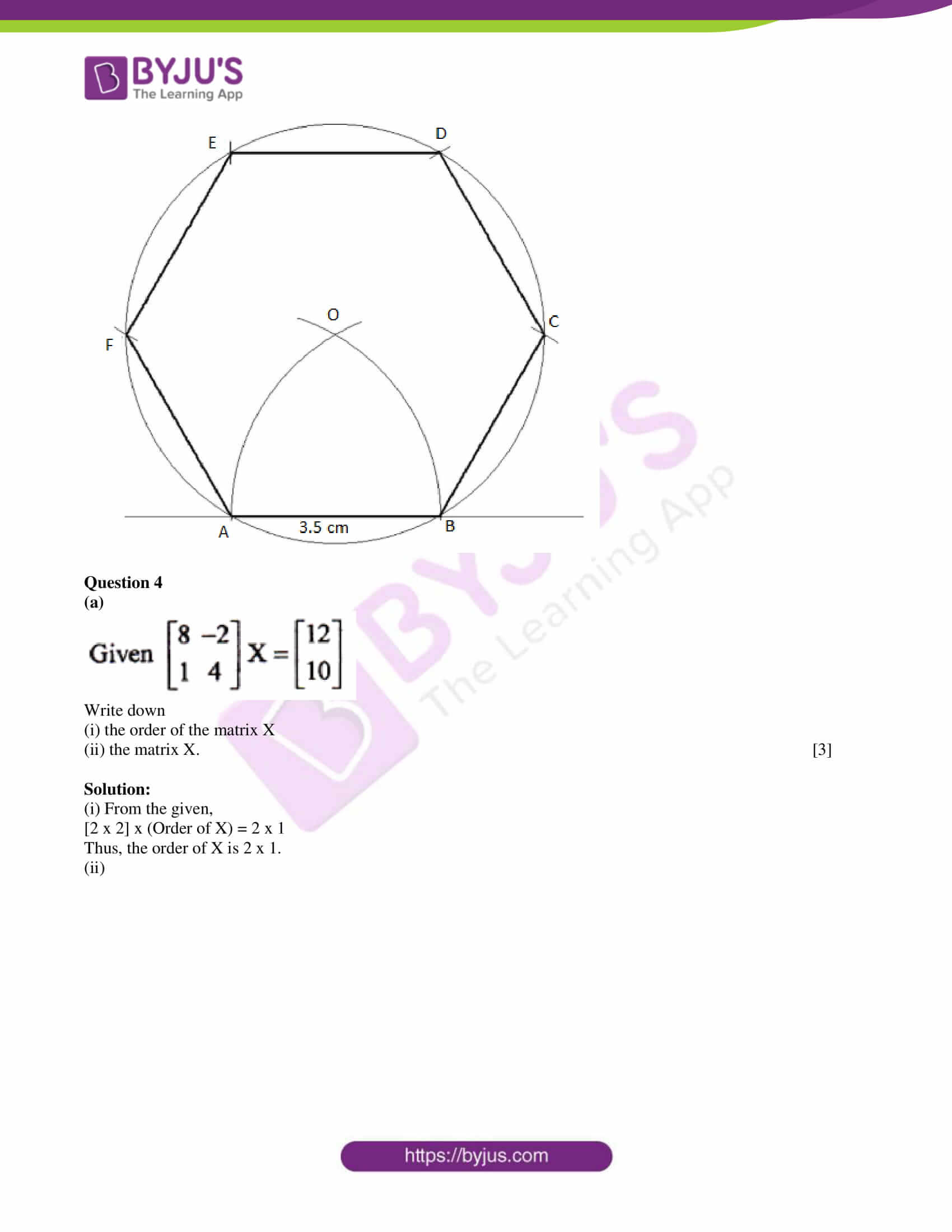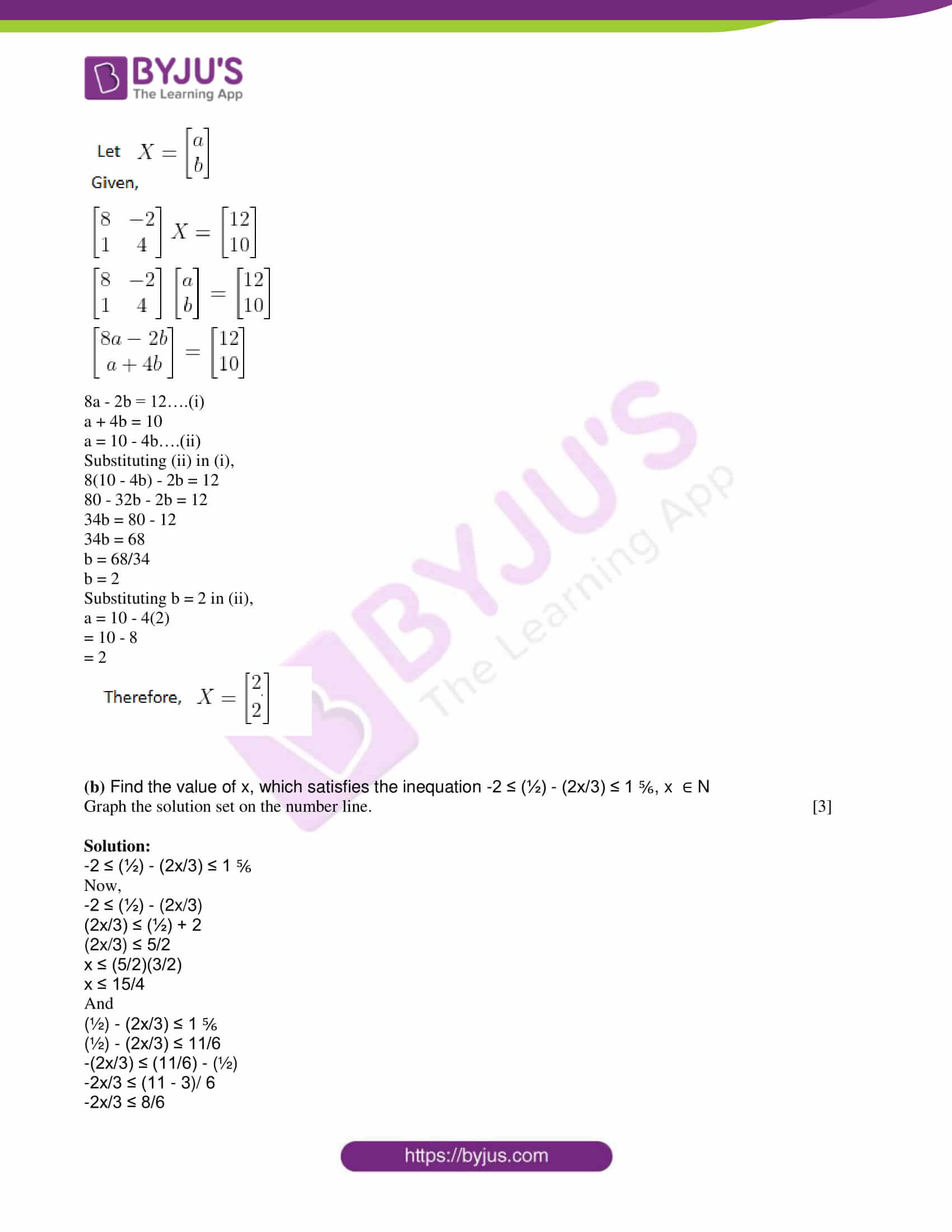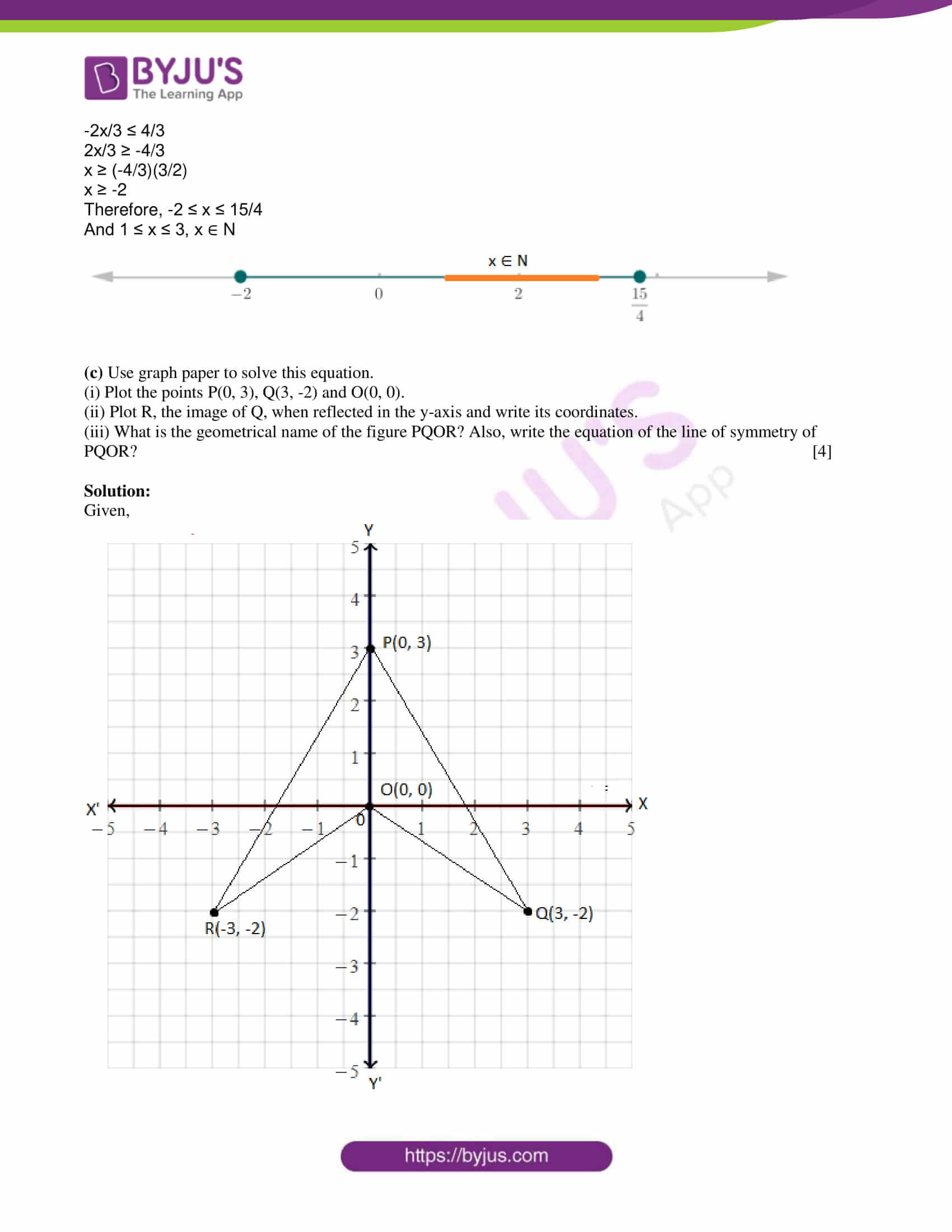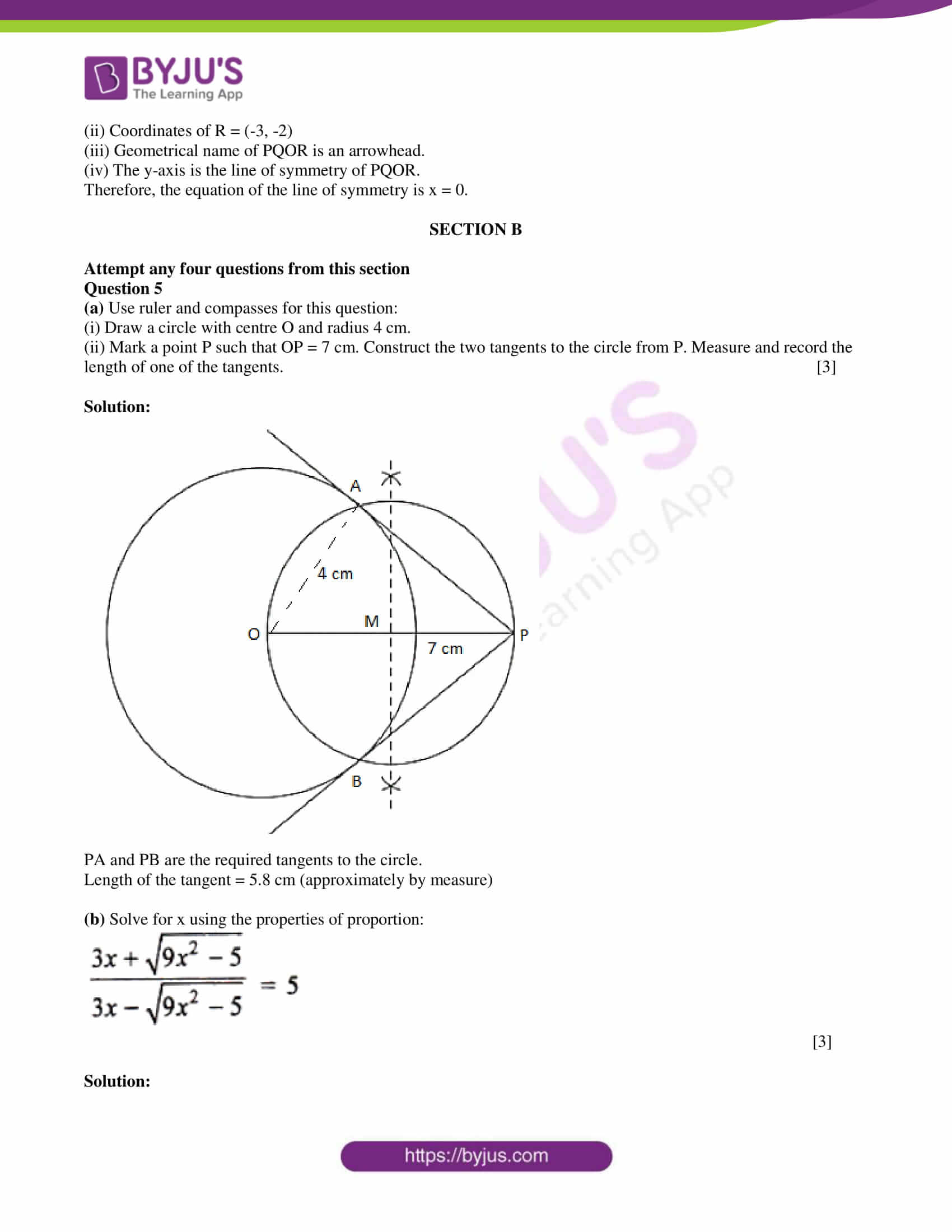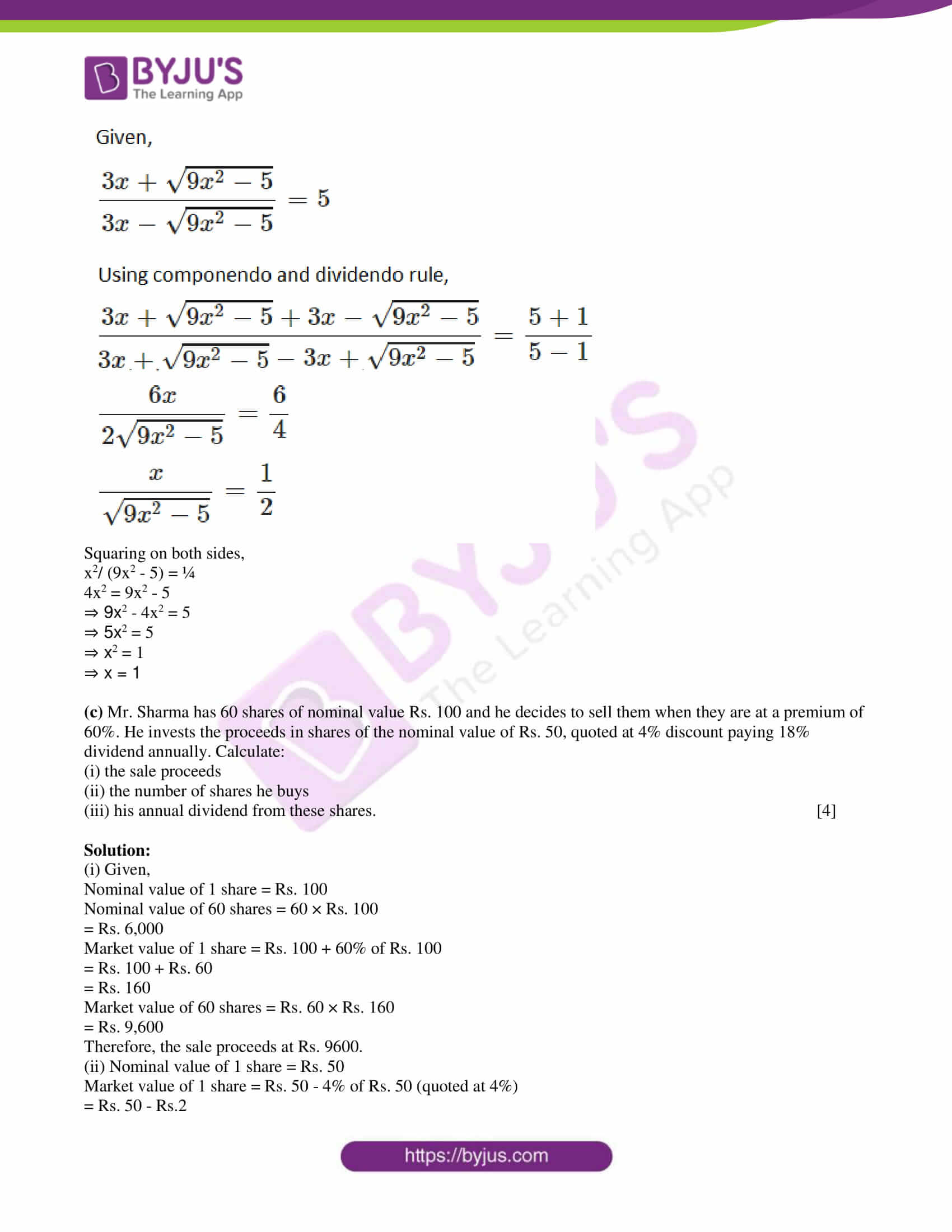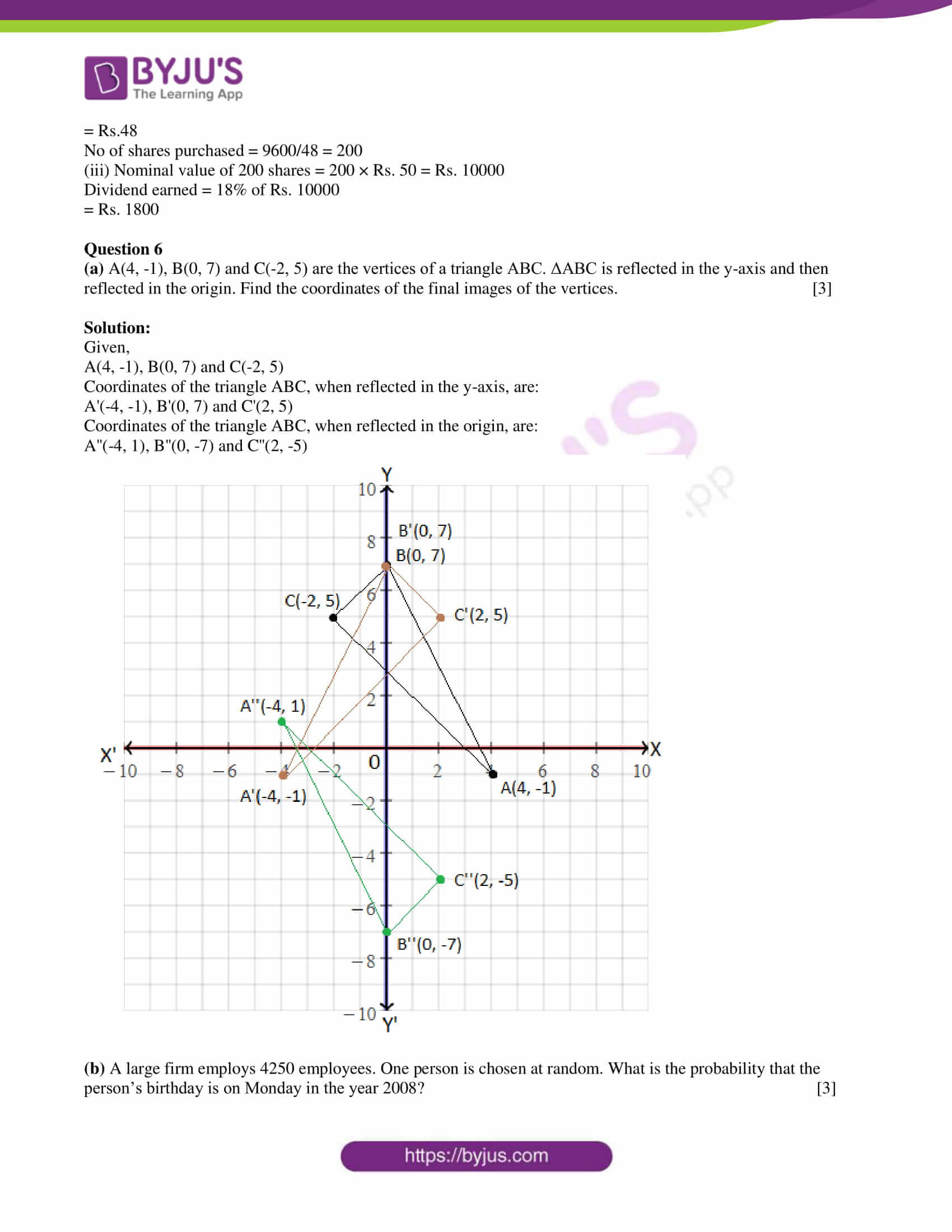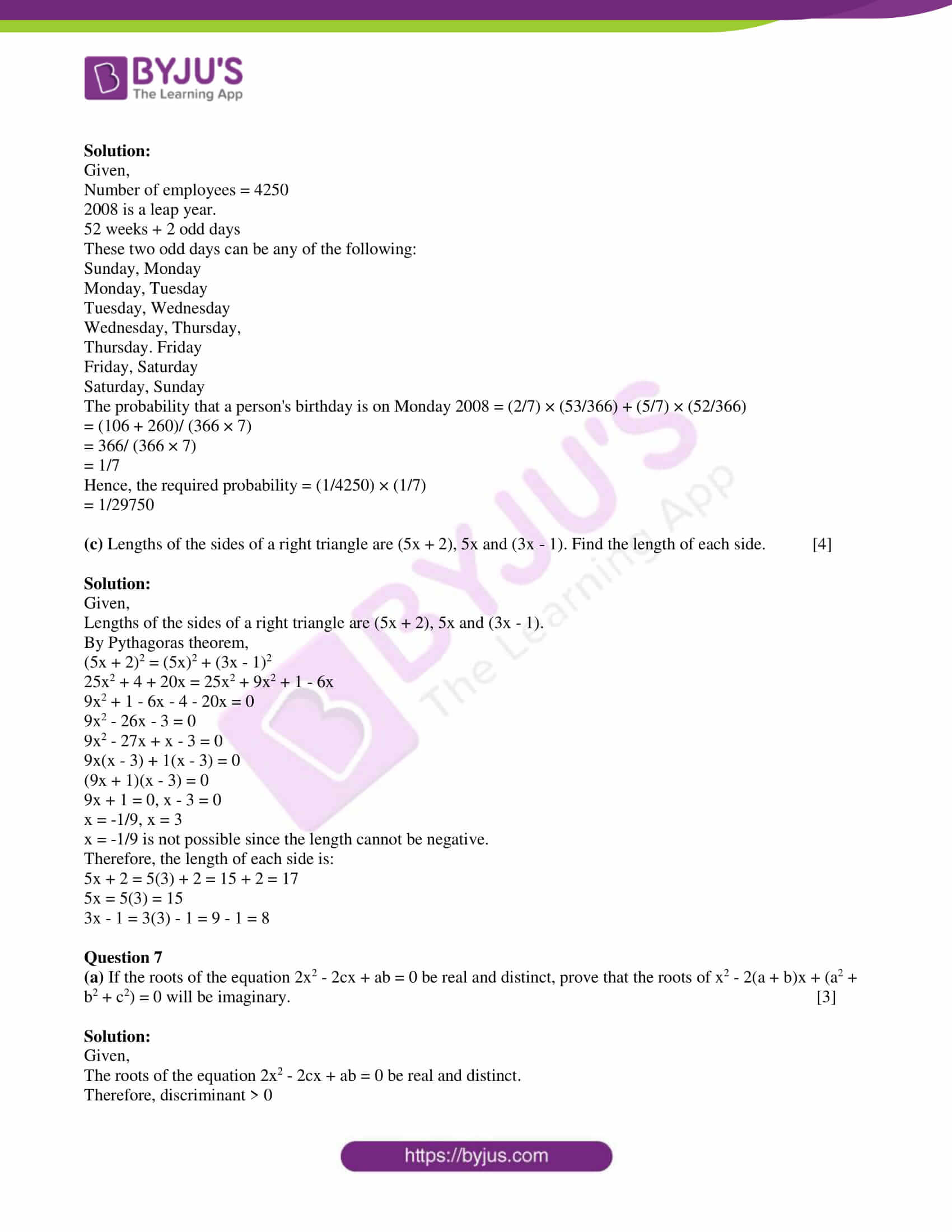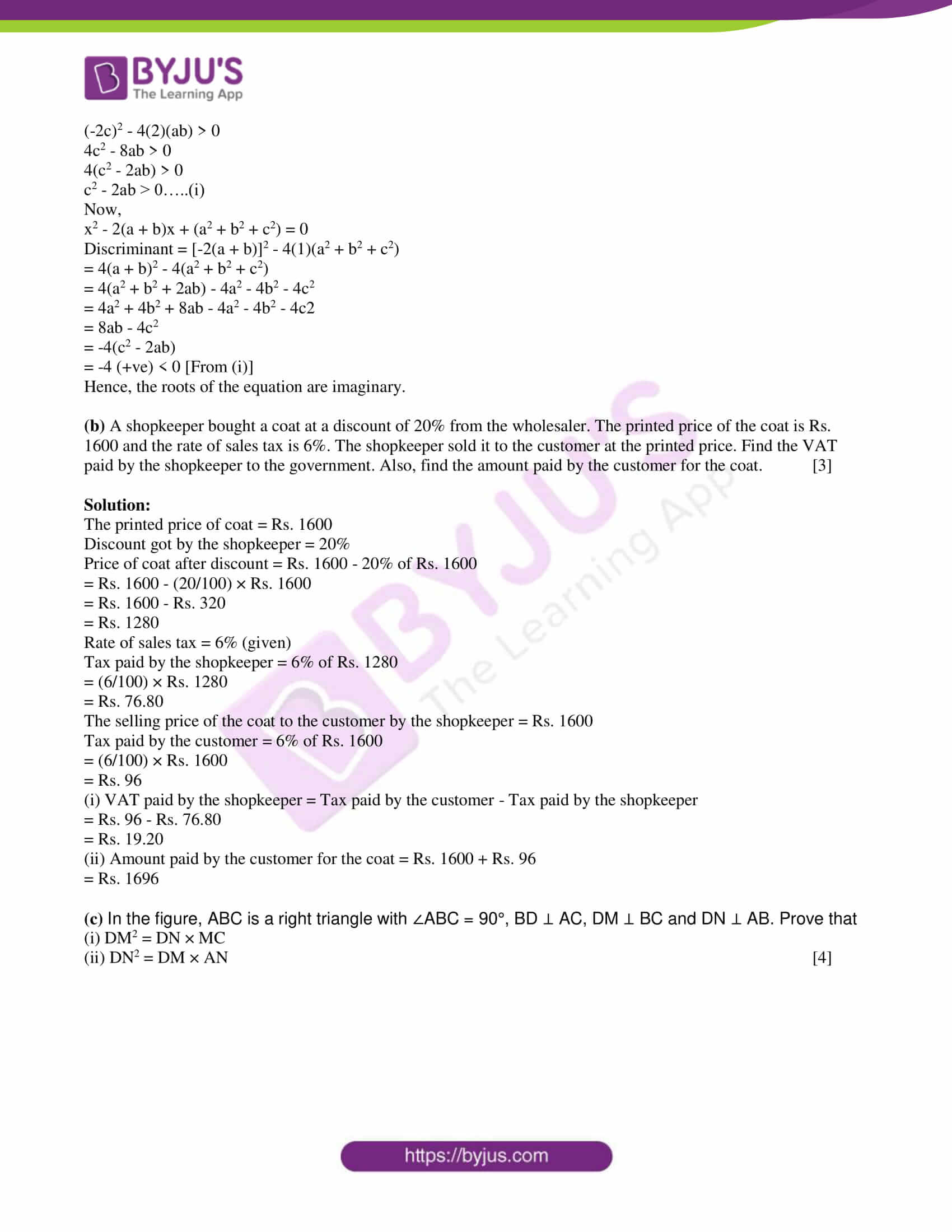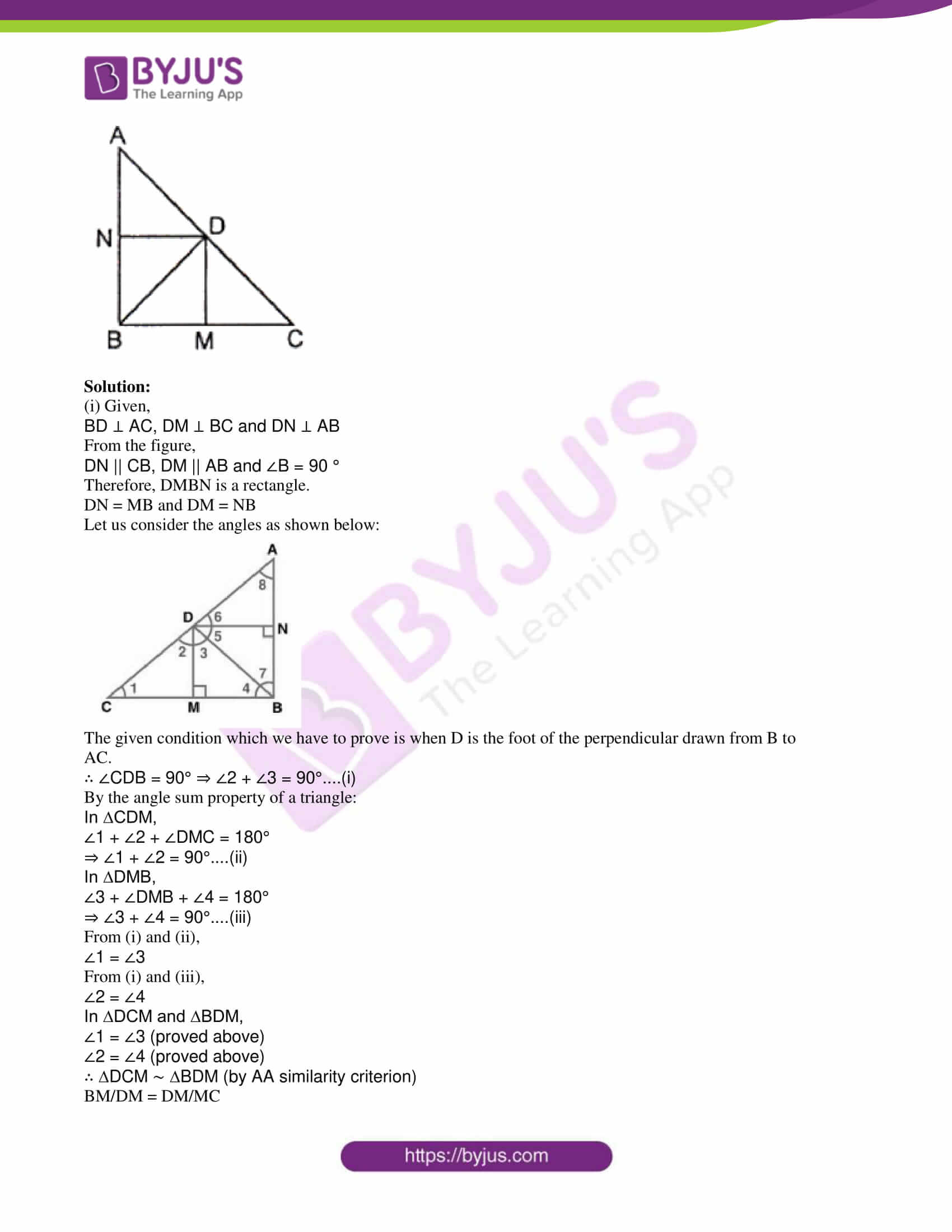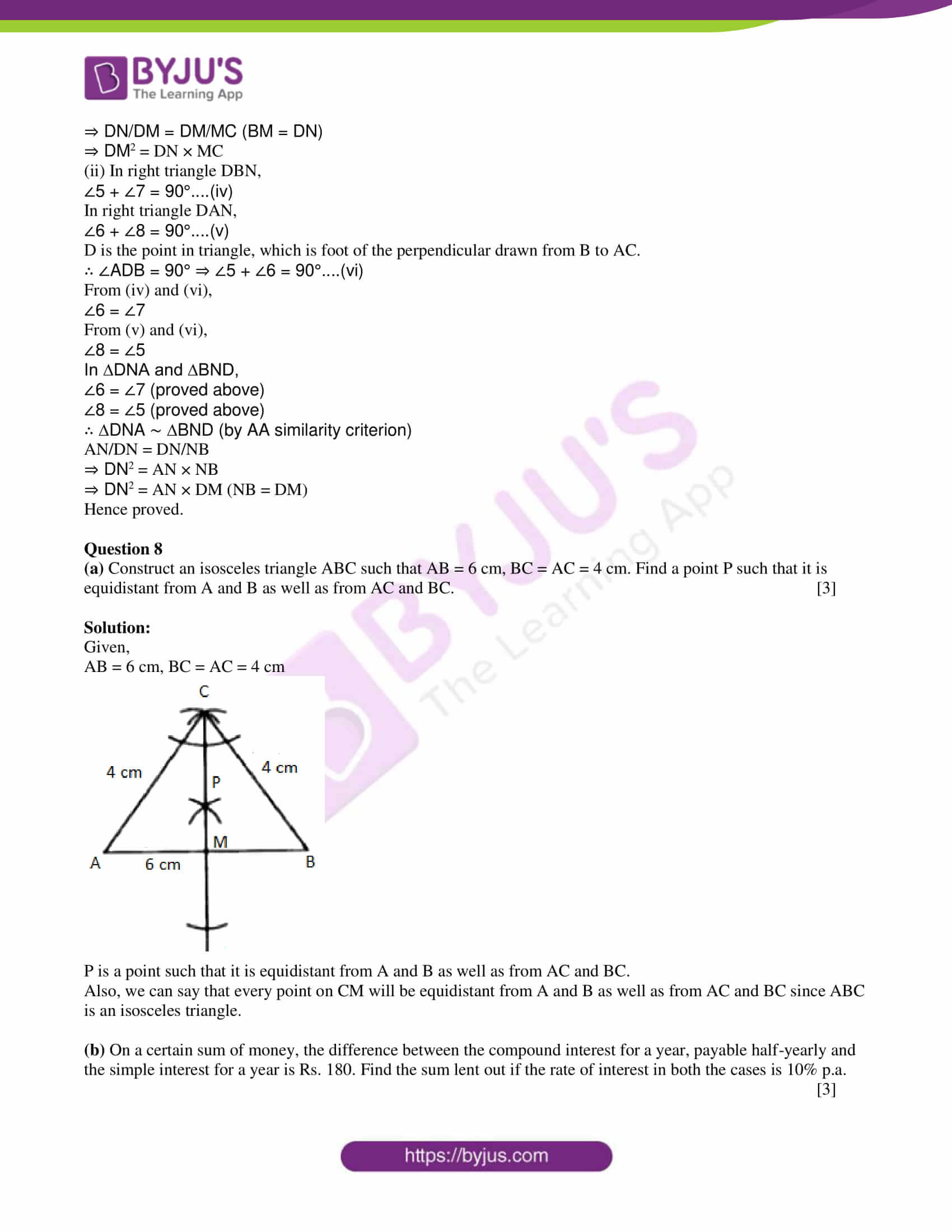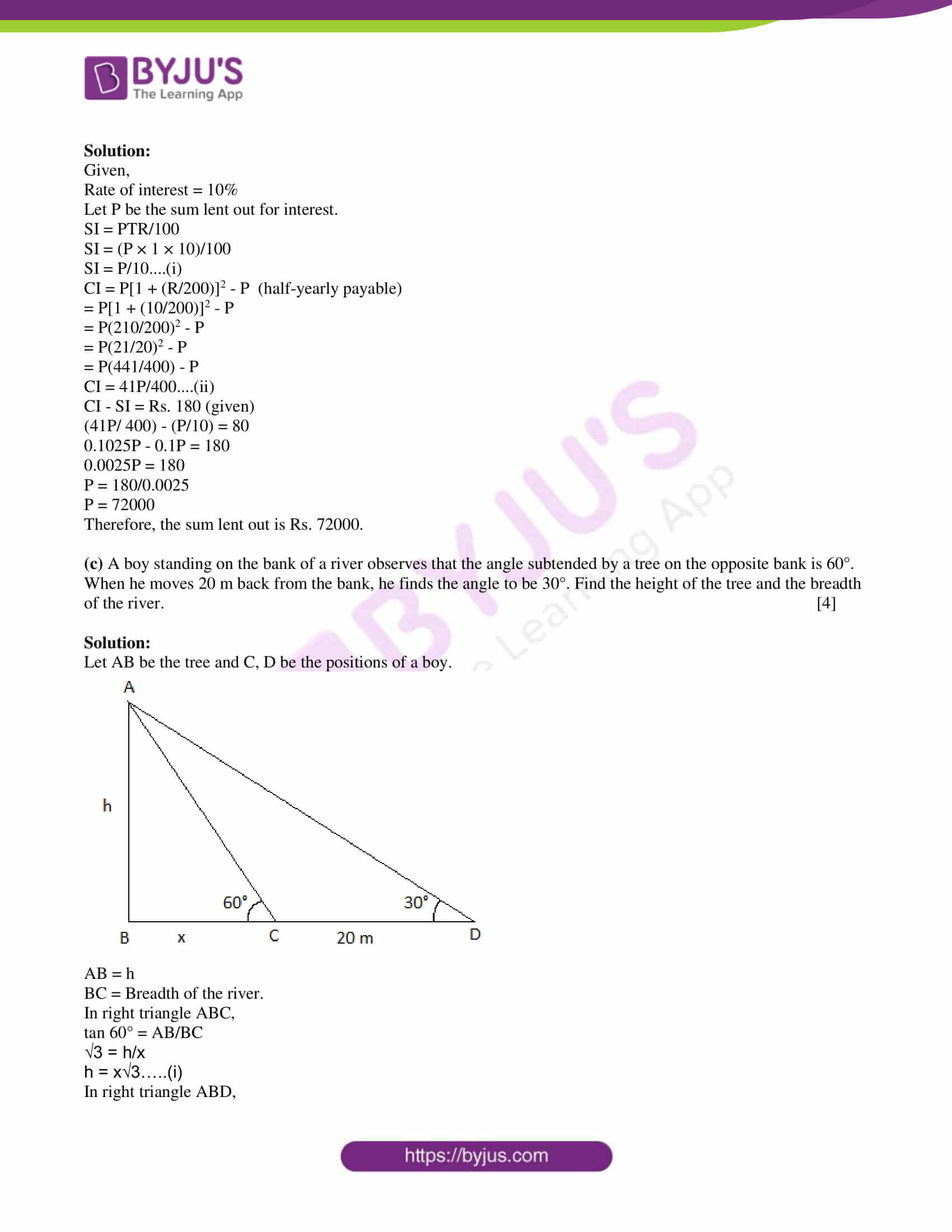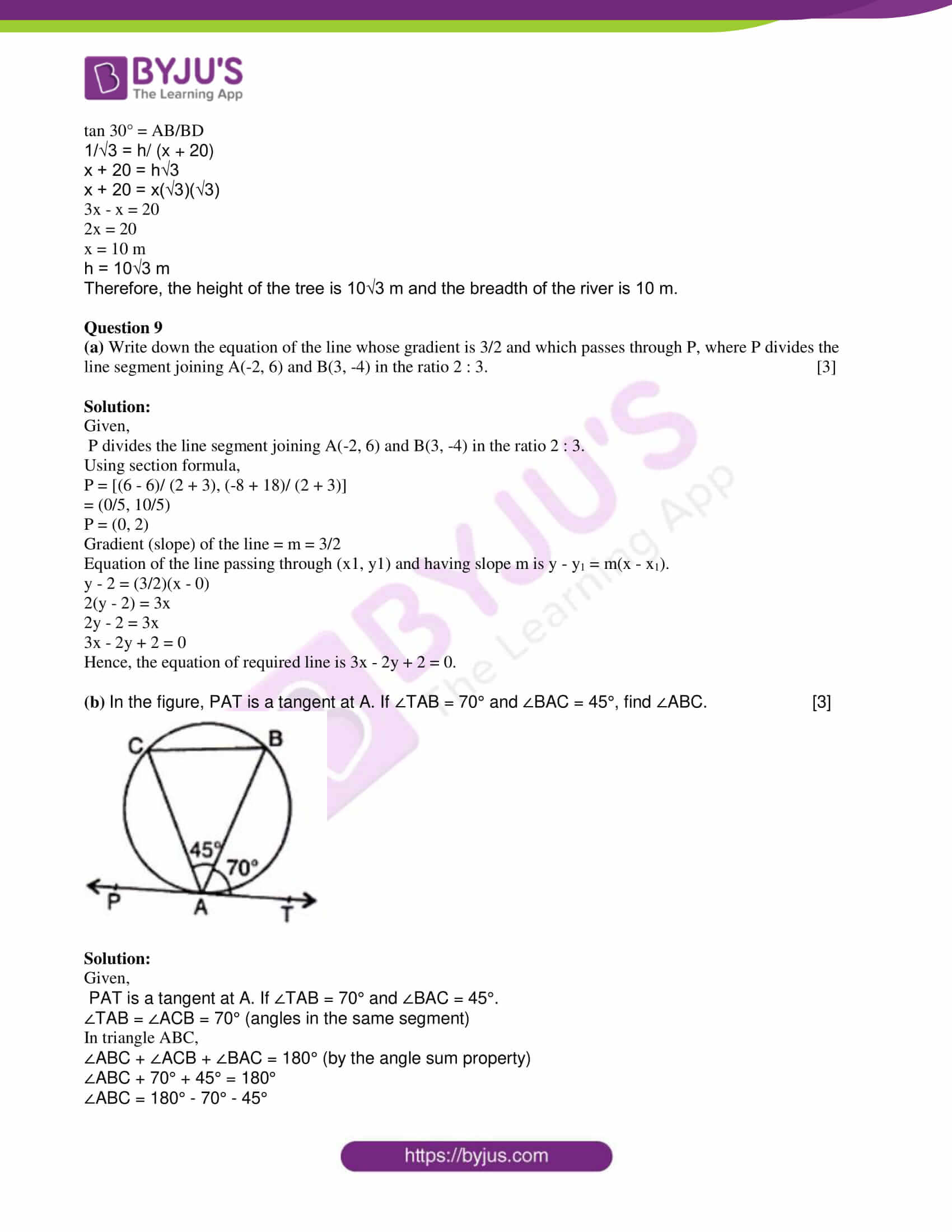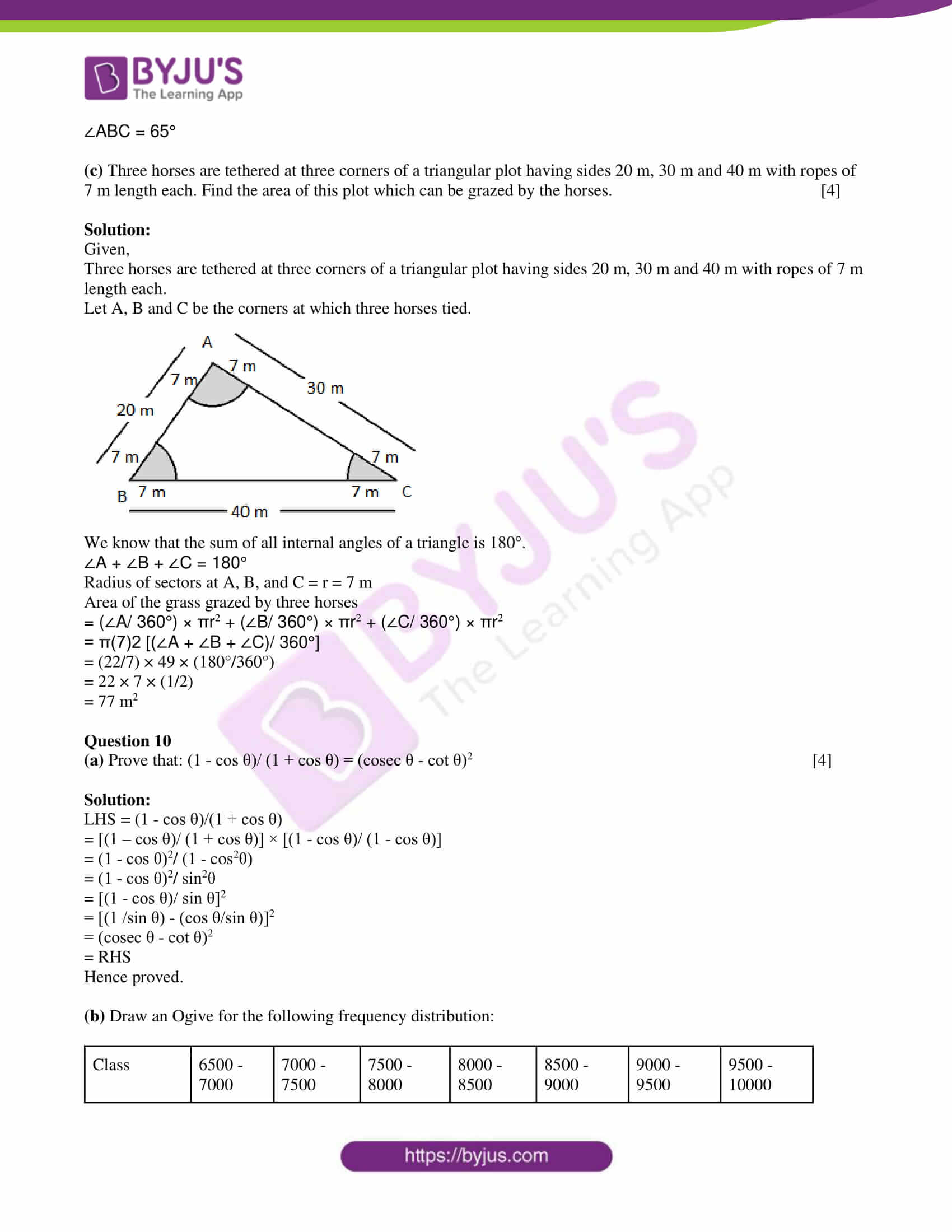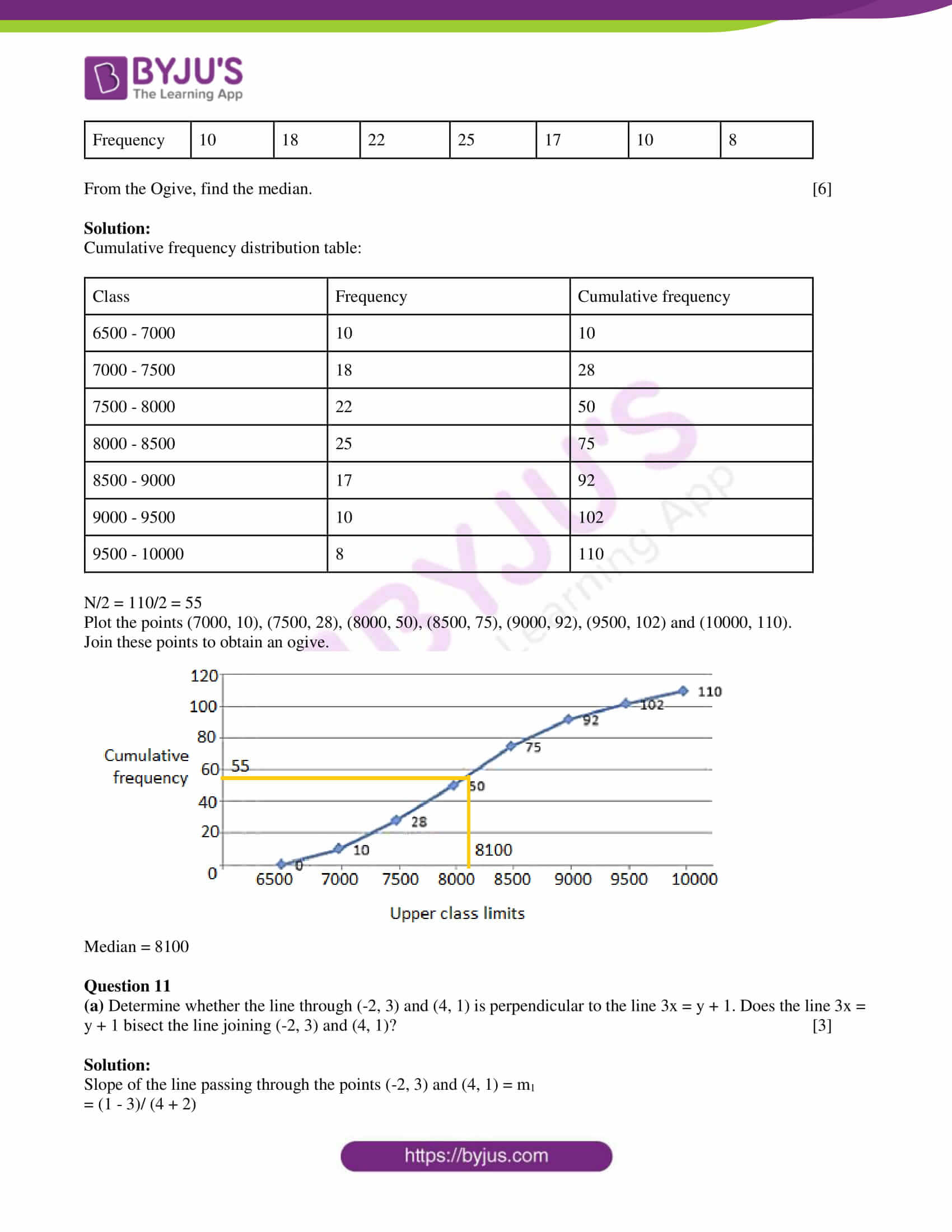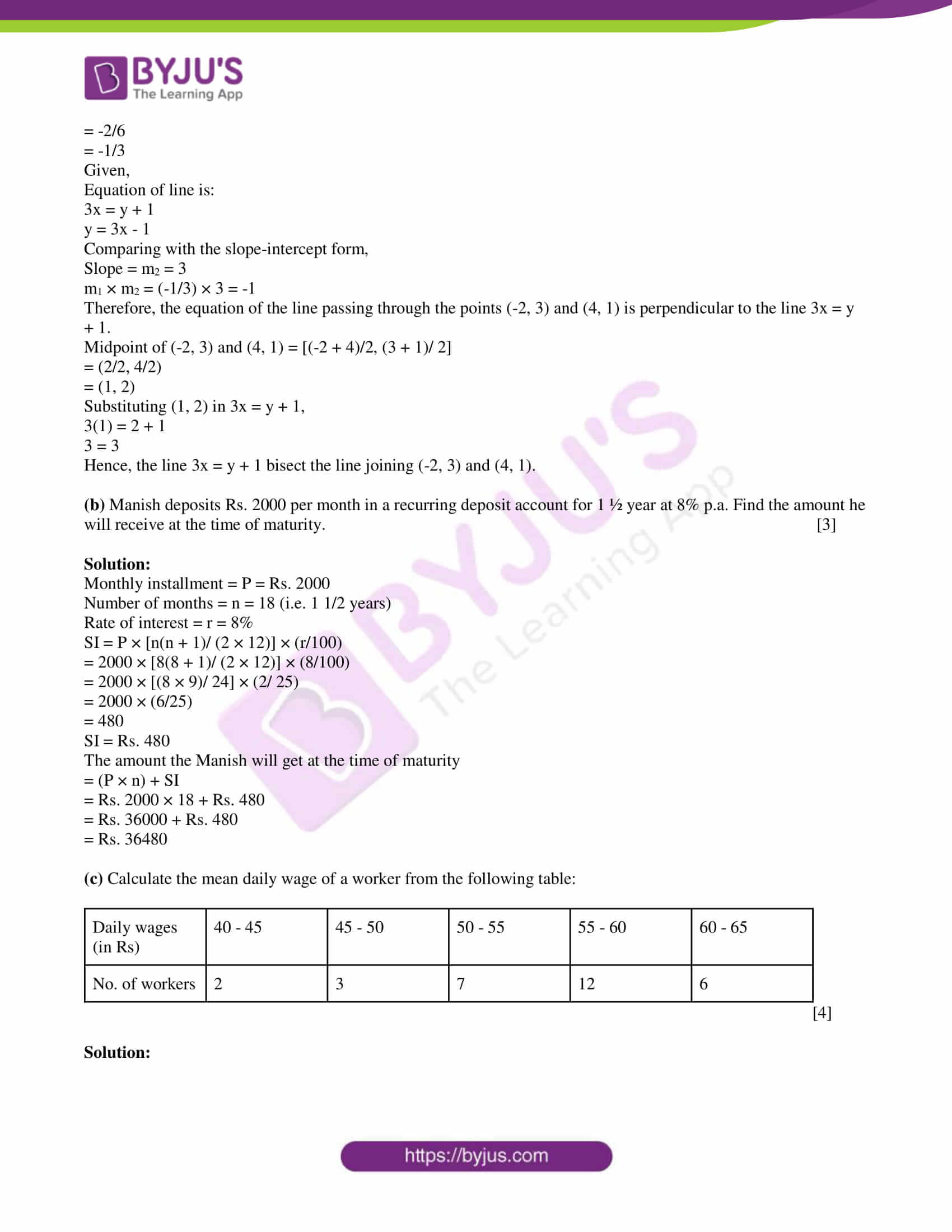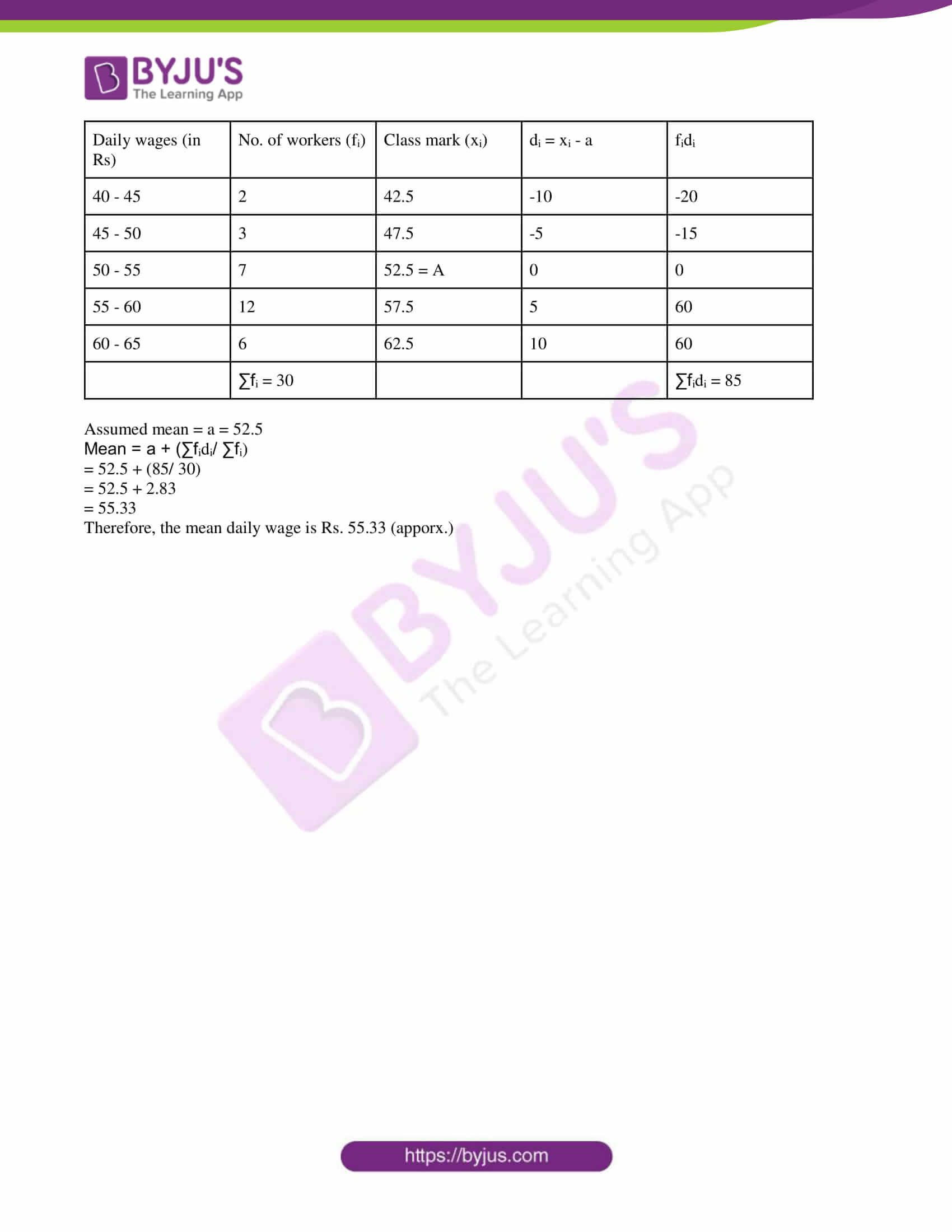SECTION A

Attempt all questions from this section.

Question 1

(a) If 2 tan θ = 5, find the value of (3 sin θ – 4 cos θ)/ (sin θ + 4 cos θ). 

Solution:

Given,

2 tan θ = 5

tan θ = 5/2

(3 sin θ – 4 cos θ)/ (sin θ + 4 cos θ)

Dividing the numerator and denominator by cos θ,

= (3 tan θ – 4)/ (tan θ + 4)

= [3(5/2) – 4)] / [(5/2) + 4]

= [(15 – 8)/ 2] / [(5 + 8)/ 2]

= 7/13

(b) Using factor theorem, show that (x – 2) is a factor of 2x3 + 5x2 – 4x – 3. 

Solution:

Let p(x) = 2x3 + 5x2 – 4x – 3

For checking (x – 2) is a factor of p(x) or not, substitute x = 2 in p(x),

p(2) = 2(2)3 + 5(2)2 – 4(2) – 3

= 2(8) + 5(4) – 8 – 3

= 16 + 20 – 11

= 25 ≠ 0

Therefore, (x – 2) is not a factor of the given polynomial 2x3 + 5x2 – 4x – 3.

(c) A chord of length 16 cm is drawn in a circle of radius 10 cm. Calculate the distance of the chord from the centre of the circle. 

Solution:

Let OA be the radius and AB be the chord of a circle.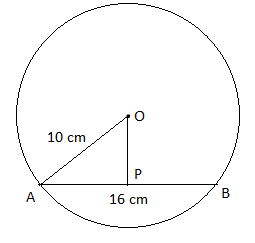OA = 10 cm

AB = 16 cm

We know that the perpendicular drawn from the centre of the circle to the chord bisects the chord.

AP = PB = 16/2 = 8 cm

In right triangle OPA,

By Pythagoras theorem,

OA2 = OP2 + AP2

(10)2 = OP2 + (8)2

OP2 = 100 – 64

OP2 = 36

OP = 6 cm

Therefore, the distance of the chord from the centre of the circle is 6 cm.

Question 2

(a) Given below are the entries in a savings bank account passbook.

 Date Particulars Withdrawal (Rs) Deposit (Rs) Balance (Rs) Jan 9, 2010 B/F _ _ 4000 Feb 20, 2010 To self 1500 _ 2500 April 15, 2010 By cash _ 1200 3700 June 15, 2010 To self 3000 _ 700 July 10, 2010 By cash _ 5000 5700

Calculate interest from Jan. to July at 4.5% per annum on minimum balance on or after 1the 10th day of each month. 

Solution:

 Month Minimum balance on or after 10th day (i.e. between 10th and the last day of the month) Jan 4000 Feb 4000 March 2500 April 2500 May 3700 June 3700 July 5700

Total = Rs. 26100

Rate of interest = R = 4.5%

Time = 7 months

I = PTR/100

= (26100 × 4.5 × 7)/ (12 × 100)

= 685.125

Hence, the interest for seven months, i.e. from Jan to July is Rs. 685.125.

(b) The circumference of the edge of a hemispherical bowl is 132 cm. Find the capacity of the bowl. 

Solution:

Let r be the radius of the hemispherical bowl.

Given,

Circumference = 132 cm

2πr = 132

2 × (22/7) × r = 132

r = (132 × 7)/ (2 × 22)

r = 21 cm

Volume of hemisphere = (2/3)πr3

= (2/3) × (22/7) × 21 × 21 × 21

= 44 × 21 × 21

= 19404 cm3

Therefore, the capacity of the hemispherical bowl is 19404 cm3.

(c) A cylindrical can whose base is horizontal and of radius 3.5 cm contains sufficient water so that when a sphere is placed in the can, the water just covers the sphere. Given that the sphere just fits into the can, calculate:

(i) the total surface area of the can in contact with water when the sphere is in it.

(ii) the depth of water in the can before the sphere was put into the can. 

Solution:

Given,

Radius of cylindrical can = r = 3.5 cm

Given that the sphere just fits into the can.

Thus, Radius of sphere = Radius of can = r = 3.5 cm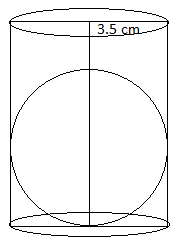Volume of cylinder up to the height 2r (diameter of sphere) of cylinder = πr2 (2r)

= 2πr3

Volume of water in the gap between sphere and cylinder = Volume of cylinder up to height 2r – Volume of sphere

= 2πr3 – (4/3)πr3

= (2/3)πr3

(i) Surface area of can which is in contact with the water when sphere is in it = Area of the base + Curved surface area of cylinder up to height 2r

= πr2 + 2πrh

= πr2 + 2πr(2r)

= 5πr2

= 5 × (22/7) × 3.5 × 3.5

= 192.5 cm2

(ii) Depth of the water in the can before the sphere is put in it = Volume of water/ Area of base of the can

= [(2/3)πr3]/ πr2

= (2/3)r

= (2/3) × 3.5

= 7/3 cm

Question 3

(a) The numbers 13, 15, 17, 18, and n are arranged in ascending order. If the mean is equal to the median, find the value of n. 

Solution:

Given,

The ascending order of numbers is:

13, 15, 17, 18, n

Mean = Sum of observations/ Number of observations

= (13 + 15 + 17 + 18 + n)/ 5

= (63 + n)/5

Number of observations = n = 5 (odd)

Median = (n + 1)/2 th observation

= (5 + 1)/2

= 6/2

3rd observation

= 17

According to the given,

Mean = Median

(63 + n)/5 = 17

63 + n = 85

n = 85 – 63

n = 22

(b) In the figure, AB is a common tangent to two circles intersecting at C and D. Write down the measure of (∠ACB + ∠ADB). Justify your answer.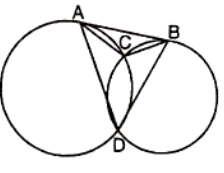Solution:

Given,

AB is a common tangent to two circles intersecting at C and D.

We know that the angle made by the chord and tangent is equal to the angles in the alternate segment.

∠CBA = ∠CDB….(i)

and

∠CAB = ∠CDA….(ii)

∠CBA + ∠CAB = ∠CDB + ∠CDA

180° – ∠ACB = ∠ADB (in triangle ABC, ∠CBA + ∠CAB + ∠ACB = 180°)

(c) Draw a regular hexagon of side 3.5 cm. Circumscribe a circle to it. 

Solution: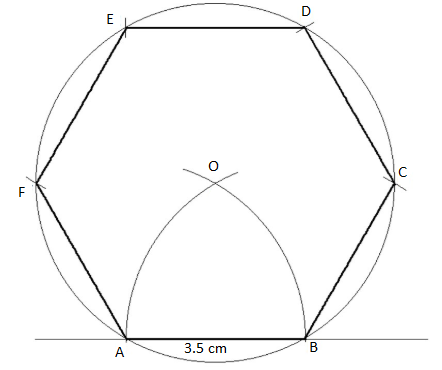Question 4

(a)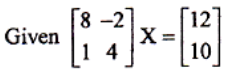Write down

(i) the order of the matrix X

(ii) the matrix X. 

Solution:

(i) From the given,

[2 x 2] x (Order of X) = 2 x 1

Thus, the order of X is 2 x 1.

(ii)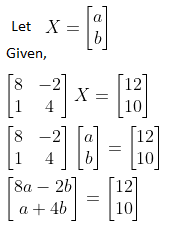8a – 2b = 12….(i)

a + 4b = 10

a = 10 – 4b….(ii)

Substituting (ii) in (i),

8(10 – 4b) – 2b = 12

80 – 32b – 2b = 12

34b = 80 – 12

34b = 68

b = 68/34

b = 2

Substituting b = 2 in (ii),

a = 10 – 4(2)

= 10 – 8

= 2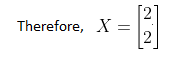(b) Find the value of x, which satisfies the inequation -2 ≤ (½) – (2x/3) ≤ 1 ⅚, x ∈ N

Graph the solution set on the number line. 

Solution:

-2 ≤ (½) – (2x/3) ≤ 1 ⅚

Now,

-2 ≤ (½) – (2x/3)

(2x/3) ≤ (½) + 2

(2x/3) ≤ 5/2

x ≤ (5/2)(3/2)

x ≤ 15/4

And

(½) – (2x/3) ≤ 1 ⅚

(½) – (2x/3) ≤ 11/6

-(2x/3) ≤ (11/6) – (½)

-2x/3 ≤ (11 – 3)/ 6

-2x/3 ≤ 8/6

-2x/3 ≤ 4/3

2x/3 ≥ -4/3

x ≥ (-4/3)(3/2)

x ≥ -2

Therefore, -2 ≤ x ≤ 15/4

And 1 ≤ x ≤ 3, x ∈ N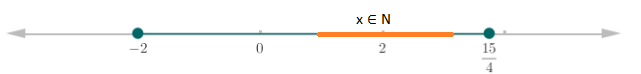(c) Use graph paper to solve this equation.

(i) Plot the points P(0, 3), Q(3, -2) and O(0, 0).

(ii) Plot R, the image of Q, when reflected in the y-axis and write its coordinates.

(iii) What is the geometrical name of the figure PQOR? Also, write the equation of the line of symmetry of PQOR? 

Solution:

Given,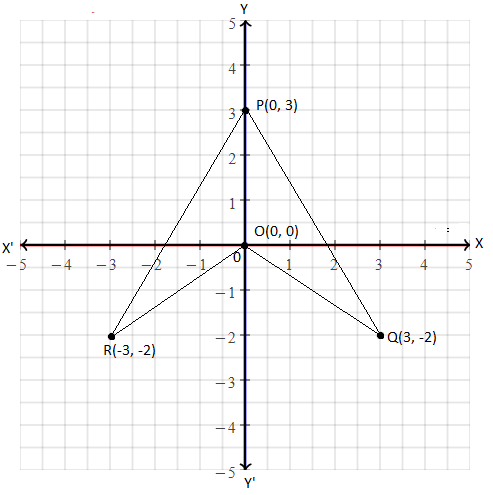(ii) Coordinates of R = (-3, -2)

(iii) Geometrical name of PQOR is an arrowhead.

(iv) The y-axis is the line of symmetry of PQOR.

Therefore, the equation of the line of symmetry is x = 0.

SECTION B

Attempt any four questions from this section

Question 5

(a) Use ruler and compasses for this question:

(i) Draw a circle with centre O and radius 4 cm.

(ii) Mark a point P such that OP = 7 cm. Construct the two tangents to the circle from P. Measure and record the length of one of the tangents. 

Solution: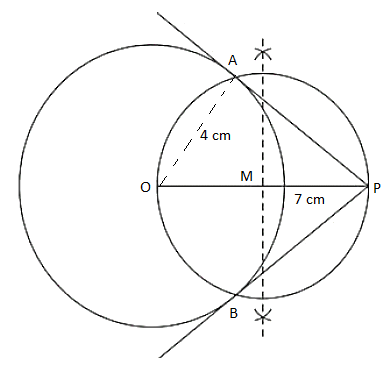PA and PB are the required tangents to the circle.

Length of the tangent = 5.8 cm (approximately by measure)

(b) Solve for x using the properties of proportion: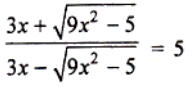Solution: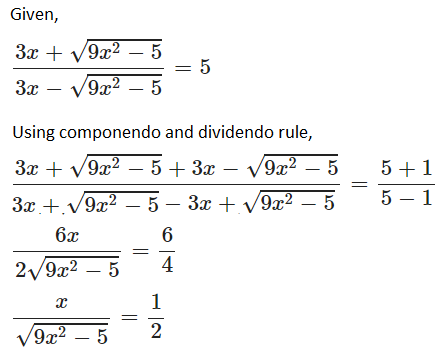Squaring on both sides,

x2/ (9x2 – 5) = ¼

4x2 = 9x2 – 5

⇒ 9x2 – 4x2 = 5

⇒ 5x2 = 5

⇒ x2 = 1

⇒ x = 1

(c) Mr. Sharma has 60 shares of nominal value Rs. 100 and he decides to sell them when they are at a premium of 60%. He invests the proceeds in shares of the nominal value of Rs. 50, quoted at 4% discount paying 18% dividend annually. Calculate:

(i) the sale proceeds

(ii) the number of shares he buys

(iii) his annual dividend from these shares 

Solution:

(i) Given,

Nominal value of 1 share = Rs. 100

Nominal value of 60 shares = 60 × Rs. 100

= Rs. 6,000

Market value of 1 share = Rs. 100 + 60% of Rs. 100

= Rs. 100 + Rs. 60

= Rs. 160

Market value of 60 shares = Rs. 60 × Rs. 160

= Rs. 9,600

Therefore, the sale proceeds at Rs. 9600.

(ii) Nominal value of 1 share = Rs. 50

Market value of 1 share = Rs. 50 – 4% of Rs. 50 (quoted at 4%)

= Rs. 50 – Rs.2

= Rs.48

No of shares purchased = 9600/48 = 200

(iii) Nominal value of 200 shares = 200 × Rs. 50 = Rs. 10000

Dividend earned = 18% of Rs. 10000

= Rs. 1800

Question 6

(a) A(4, -1), B(0, 7) and C(-2, 5) are the vertices of a triangle ABC. ΔABC is reflected in the y-axis and then reflected in the origin. Find the coordinates of the final images of the vertices. 

Solution:

Given,

A(4, -1), B(0, 7) and C(-2, 5)

Coordinates of the triangle ABC, when reflected in the y-axis, are:

A'(-4, -1), B'(0, 7) and C'(2, 5)

Coordinates of the triangle ABC, when reflected in the origin, are:

A”(-4, 1), B”(0, -7) and C”(2, -5)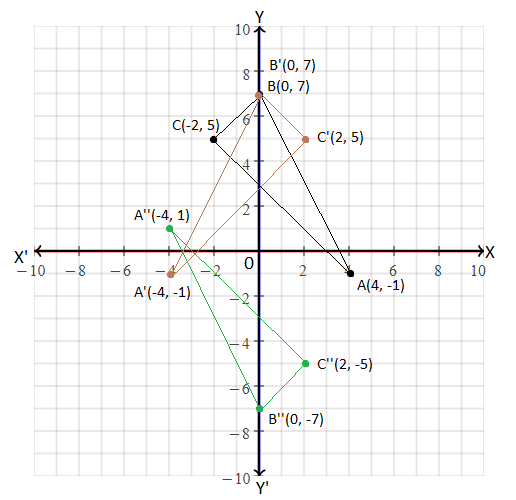(b) A large firm employs 4250 employees. One person is chosen at random. What is the probability that the person’s birthday is on Monday in the year 2008? 

Solution:

Given,

Number of employees = 4250

2008 is a leap year.

52 weeks + 2 odd days

These two odd days can be any of the following:

Sunday, Monday

Monday, Tuesday

Tuesday, Wednesday

Wednesday, Thursday,

Thursday. Friday

Friday, Saturday

Saturday, Sunday

The probability that a person’s birthday is on Monday 2008 = (2/7) × (53/366) + (5/7) × (52/366)

= (106 + 260)/ (366 × 7)

= 366/ (366 × 7)

= 1/7

Hence, the required probability = (1/4250) × (1/7)

= 1/29750

(c) Lengths of the sides of a right triangle are (5x + 2), 5x and (3x – 1). Find the length of each side. 

Solution:

Given,

Lengths of the sides of a right triangle are (5x + 2), 5x and (3x – 1).

By Pythagoras theorem,

(5x + 2)2 = (5x)2 + (3x – 1)2

25x2 + 4 + 20x = 25x2 + 9x2 + 1 – 6x

9x2 + 1 – 6x – 4 – 20x = 0

9x2 – 26x – 3 = 0

9x2 – 27x + x – 3 = 0

9x(x – 3) + 1(x – 3) = 0

(9x + 1)(x – 3) = 0

9x + 1 = 0, x – 3 = 0

x = -1/9, x = 3

x = -1/9 is not possible since the length cannot be negative.

Therefore, the length of each side is:

5x + 2 = 5(3) + 2 = 15 + 2 = 17

5x = 5(3) = 15

3x – 1 = 3(3) – 1 = 9 – 1 = 8

Question 7

(a) If the roots of the equation 2x2 – 2cx + ab = 0 be real and distinct, prove that the roots of x2 – 2(a + b)x + (a2 + b2 + c2) = 0 will be imaginary. 

Solution:

Given,

The roots of the equation 2x2 – 2cx + ab = 0 be real and distinct.

Therefore, discriminant > 0

(-2c)2 – 4(2)(ab) > 0

4c2 – 8ab > 0

4(c2 – 2ab) > 0

c2 – 2ab > 0…..(i)

Now,

x2 – 2(a + b)x + (a2 + b2 + c2) = 0

Discriminant = [-2(a + b)]2 – 4(1)(a2 + b2 + c2)

= 4(a + b)2 – 4(a2 + b2 + c2)

= 4(a2 + b2 + 2ab) – 4a2 – 4b2 – 4c2

= 4a2 + 4b2 + 8ab – 4a2 – 4b2 – 4c2

= 8ab – 4c2

= -4(c2 – 2ab)

= -4 (+ve) < 0 [From (i)]

Hence, the roots of the equation are imaginary.

(b) A shopkeeper bought a coat at a discount of 20% from the wholesaler. The printed price of the coat is Rs. 1600 and the rate of sales tax is 6%. The shopkeeper sold it to the customer at the printed price. Find the VAT paid by the shopkeeper to the government. Also, find the amount paid by the customer for the coat. 

Solution:

The printed price of coat = Rs. 1600

Discount got by the shopkeeper = 20%

Price of coat after discount = Rs. 1600 – 20% of Rs. 1600

= Rs. 1600 – (20/100) × Rs. 1600

= Rs. 1600 – Rs. 320

= Rs. 1280

Rate of sales tax = 6% (given)

Tax paid by the shopkeeper = 6% of Rs. 1280

= (6/100) × Rs. 1280

= Rs. 76.80

The selling price of the coat to the customer by the shopkeeper = Rs. 1600

Tax paid by the customer = 6% of Rs. 1600

= (6/100) × Rs. 1600

= Rs. 96

(i) VAT paid by the shopkeeper = Tax paid by the customer – Tax paid by the shopkeeper

= Rs. 96 – Rs. 76.80

= Rs. 19.20

(ii) Amount paid by the customer for the coat = Rs. 1600 + Rs. 96

= Rs. 1696

(c) In the figure, ABC is a right triangle with ∠ABC = 90°, BD ⊥ AC, DM ⊥ BC and DN ⊥ AB. Prove that

(i) DM2 = DN × MC

(ii) DN2 = DM × AN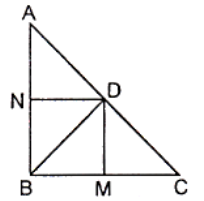Solution:

(i) Given,

BD ⊥ AC, DM ⊥ BC and DN ⊥ AB

From the figure,

DN || CB, DM || AB and ∠B = 90 °

Therefore, DMBN is a rectangle.

DN = MB and DM = NB

Let us consider the angles as shown below: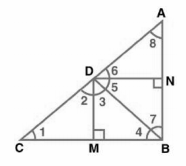The given condition which we have to prove is when D is the foot of the perpendicular drawn from B to

AC.

∴ ∠CDB = 90° ⇒ ∠2 + ∠3 = 90°….(i)

By the angle sum property of a triangle:

In ∆CDM,

∠1 + ∠2 + ∠DMC = 180°

⇒ ∠1 + ∠2 = 90°….(ii)

In ∆DMB,

∠3 + ∠DMB + ∠4 = 180°

⇒ ∠3 + ∠4 = 90°….(iii)

From (i) and (ii),

∠1 = ∠3

From (i) and (iii),

∠2 = ∠4

In ∆DCM and ∆BDM,

∠1 = ∠3 (proved above)

∠2 = ∠4 (proved above)

∴ ∆DCM ∼ ∆BDM (by AA similarity criterion)

BM/DM = DM/MC

⇒ DN/DM = DM/MC (BM = DN)

⇒ DM2 = DN × MC

(ii) In right triangle DBN,

∠5 + ∠7 = 90°….(iv)

In right triangle DAN,

∠6 + ∠8 = 90°….(v)

D is the point in the triangle, which is the foot of the perpendicular drawn from B to AC.

∴ ∠ADB = 90° ⇒ ∠5 + ∠6 = 90°….(vi)

From (iv) and (vi),

∠6 = ∠7

From (v) and (vi),

∠8 = ∠5

In ∆DNA and ∆BND,

∠6 = ∠7 (proved above)

∠8 = ∠5 (proved above)

∴ ∆DNA ∼ ∆BND (by AA similarity criterion)

AN/DN = DN/NB

⇒ DN2 = AN × NB

⇒ DN2 = AN × DM (NB = DM)

Hence proved.

Question 8

(a) Construct an isosceles triangle ABC such that AB = 6 cm, BC = AC = 4 cm. Find a point P such that it is equidistant from A and B as well as from AC and BC. 

Solution:

Given,

AB = 6 cm, BC = AC = 4 cm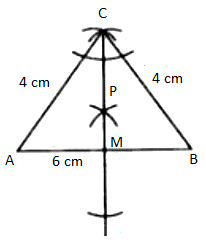P is a point such that it is equidistant from A and B as well as from AC and BC.

Also, we can say that every point on CM will be equidistant from A and B as well as from AC and BC since ABC is an isosceles triangle.

(b) On a certain sum of money, the difference between the compound interest for a year, payable half-yearly and the simple interest for a year is Rs. 180. Find the sum lent out if the rate of interest in both the cases is 10% p.a. 

Solution:

Given,

Rate of interest = 10%

Let P be the sum lent out for interest.

SI = PTR/100

SI = (P × 1 × 10)/100

SI = P/10….(i)

CI = P[1 + (R/200)]2 – P (half-yearly payable)

= P[1 + (10/200)]2 – P

= P(210/200)2 – P

= P(21/20)2 – P

= P(441/400) – P

CI = 41P/400….(ii)

CI – SI = Rs. 180 (given)

(41P/ 400) – (P/10) = 80

0.1025P – 0.1P = 180

0.0025P = 180

P = 180/0.0025

P = 72000

Therefore, the sum lent out is Rs. 72000.

(c) A boy standing on the bank of a river observes that the angle subtended by a tree on the opposite bank is 60°. When he moves 20 m back from the bank, he finds the angle to be 30°. Find the height of the tree and the breadth of the river. 

Solution:

Let AB be the tree and C, D be the positions of a boy.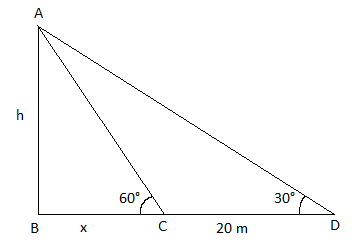AB = h

BC = Breadth of the river.

In right triangle ABC,

tan 60° = AB/BC

√3 = h/x

h = x√3…..(i)

In right triangle ABD,

tan 30° = AB/BD

1/√3 = h/ (x + 20)

x + 20 = h√3

x + 20 = x(√3)(√3)

3x – x = 20

2x = 20

x = 10 m

h = 10√3 m

Therefore, the height of the tree is 10√3 m and the breadth of the river is 10 m.

Question 9

(a) Write down the equation of the line whose gradient is 3/2 and which passes through P, where P divides the line segment joining A(-2, 6) and B(3, -4) in the ratio 2 : 3. 

Solution:

Given,

P divides the line segment joining A(-2, 6) and B(3, -4) in the ratio 2 : 3.

Using section formula,

P = [(6 – 6)/ (2 + 3), (-8 + 18)/ (2 + 3)]

= (0/5, 10/5)

P = (0, 2)

Gradient (slope) of the line = m = 3/2

Equation of the line passing through (x1, y1) and having slope m is y – y1 = m(x – x1).

y – 2 = (3/2)(x – 0)

2(y – 2) = 3x

2y – 2 = 3x

3x – 2y + 2 = 0

Hence, the equation of required line is 3x – 2y + 2 = 0.

(b) In the figure, PAT is a tangent at A. If ∠TAB = 70° and ∠BAC = 45°, find ∠ABC.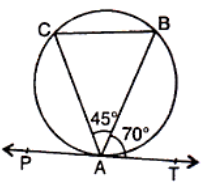Solution:

Given,

PAT is a tangent at A. If ∠TAB = 70° and ∠BAC = 45°.

∠TAB = ∠ACB = 70° (angles in the same segment)

In triangle ABC,

∠ABC + ∠ACB + ∠BAC = 180° (by the angle sum property)

∠ABC + 70° + 45° = 180°

∠ABC = 180° – 70° – 45°

∠ABC = 65°

(c) Three horses are tethered at three corners of a triangular plot having sides 20 m, 30 m and 40 m with ropes of 7 m length each. Find the area of this plot which can be grazed by the horses. 

Solution:

Given,

Three horses are tethered at three corners of a triangular plot having sides 20 m, 30 m and 40 m with ropes of 7 m length each.

Let A, B and C be the corners at which three horses tied.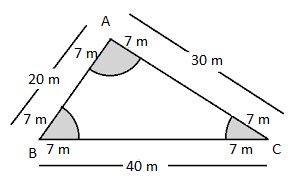We know that the sum of all internal angles of a triangle is 180°.

∠A + ∠B + ∠C = 180°

Radius of sectors at A, B, and C = r = 7 m

Area of the grass grazed by three horses

= (∠A/ 360°) × πr2 + (∠B/ 360°) × πr2 + (∠C/ 360°) × πr2

= π(7)2 [(∠A + ∠B + ∠C)/ 360°]

= (22/7) × 49 × (180°/360°)

= 22 × 7 × (1/2)

= 77 m2

Question 10

(a) Prove that: (1 – cos θ)/ (1 + cos θ) = (cosec θ – cot θ)2 

Solution:

LHS = (1 – cos θ)/(1 + cos θ)

= [(1 – cos θ)/ (1 + cos θ)] × [(1 – cos θ)/ (1 – cos θ)]

= (1 – cos θ)2/ (1 – cos2θ)

= (1 – cos θ)2/ sin2θ

= [(1 – cos θ)/ sin θ]2

= [(1 /sin θ) – (cos θ/sin θ)]2

= (cosec θ – cot θ)2

= RHS

Hence proved.

(b) Draw an Ogive for the following frequency distribution:

 Class 6500 – 7000 7000 – 7500 7500 – 8000 8000 – 8500 8500 – 9000 9000 – 9500 9500 – 10000 Frequency 10 18 22 25 17 10 8

From the Ogive, find the median. 

Solution:

Cumulative frequency distribution table:

 Class Frequency Cumulative frequency 6500 – 7000 10 10 7000 – 7500 18 28 7500 – 8000 22 50 8000 – 8500 25 75 8500 – 9000 17 92 9000 – 9500 10 102 9500 – 10000 8 110

N/2 = 110/2 = 55

Plot the points (7000, 10), (7500, 28), (8000, 50), (8500, 75), (9000, 92), (9500, 102) and (10000, 110).

Join these points to obtain an ogive.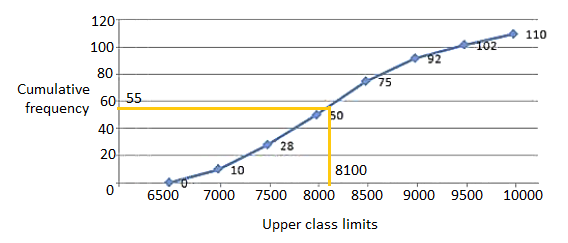Median = 8100

Question 11

(a) Determine whether the line through (-2, 3) and (4, 1) is perpendicular to the line 3x = y + 1. Does the line 3x = y + 1 bisect the line joining (-2, 3) and (4, 1)? 

Solution:

Slope of the line passing through the points (-2, 3) and (4, 1) = m1

= (1 – 3)/ (4 + 2)

= -2/6

= -1/3

Given,

Equation of line is:

3x = y + 1

y = 3x – 1

Comparing with the slope-intercept form,

Slope = m2 = 3

m1 × m2 = (-1/3) × 3 = -1

Therefore, the equation of the line passing through the points (-2, 3) and (4, 1) is perpendicular to the line 3x = y + 1.

Midpoint of (-2, 3) and (4, 1) = [(-2 + 4)/2, (3 + 1)/ 2]

= (2/2, 4/2)

= (1, 2)

Substituting (1, 2) in 3x = y + 1,

3(1) = 2 + 1

3 = 3

Hence, the line 3x = y + 1 bisect the line joining (-2, 3) and (4, 1).

(b) Manish deposits Rs. 2000 per month in a recurring deposit account for 1 ½ year at 8% p.a. Find the amount he will receive at the time of maturity. 

Solution:

Monthly installment = P = Rs. 2000

Number of months = n = 18 (i.e. 1 1/2 years)

Rate of interest = r = 8%

SI = P × [n(n + 1)/ (2 × 12)] × (r/100)

= 2000 × [8(8 + 1)/ (2 × 12)] × (8/100)

= 2000 × [(8 × 9)/ 24] × (2/ 25)

= 2000 × (6/25)

= 480

SI = Rs. 480

The amount Manish will get at the time of maturity

= (P × n) + SI

= Rs. 2000 × 18 + Rs. 480

= Rs. 36000 + Rs. 480

= Rs. 36480

(c) Calculate the mean daily wage of a worker from the following table:

 Daily wages (in Rs) 40 – 45 45 – 50 50 – 55 55 – 60 60 – 65 No. of workers 2 3 7 12 6


Solution:

 Daily wages (in Rs) No. of workers (fi) Class mark (xi) di = xi – a fidi 40 – 45 2 42.5 -10 -20 45 – 50 3 47.5 -5 -15 50 – 55 7 52.5 = A 0 0 55 – 60 12 57.5 5 60 60 – 65 6 62.5 10 60 ∑fi = 30 ∑fidi = 85

Assumed mean = a = 52.5

Mean = a + (∑fidi/ ∑fi)

= 52.5 + (85/ 30)

= 52.5 + 2.83

= 55.33

Therefore, the mean daily wage is Rs. 55.33 (apporx.)